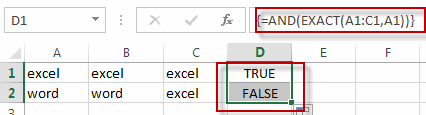# Check If Multiple Cells are Equal In Excel

This post will guide you how to check if the values of multiple cells are equal with a formula in Excel. How do I verify that multiple cellsare the same in Excel. How to compare three or more cells in Excel to see if they are the same with a formula.

## Check If Multiple Cells are Equal

Assuming that you have a list of data in range A1:C1, and you want to compare if these cells are equal, if so, then return True, otherwise, return False. How to achieve it.
You need to create an Excel array formula based on the AND function and the EXACT function. Just like this:

`=AND(EXACT(A1:C1,A1))`

Type this formula into a blank cell, and press Ctrl +Shift +Enter shortcuts in your keyboard.

###There is another formula based on the COUNTIF function to achieve the same result. Like this:

`=COUNTIF(A1:C1,A1)=3`

### Related Functions

• Excel AND function
The Excel AND function returns TRUE if all of arguments are TRUE, and it returns FALSE if any of arguments are FALSE.The syntax of the AND function is as below:= AND (condition1,[condition2],…) …
• Excel COUNTIF function
The Excel COUNTIF function will count the number of cells in a range that meet a given criteria. This function can be used to count the different kinds of cells with number, date, text values, blank, non-blanks, or containing specific characters.etc.= COUNTIF (range, criteria)…
• Excel EXACT function
The Excel EXACT function compares if two text strings are the same and returns TRUE if they are the same, Or, it will return FALSE.The syntax of the EXACT function is as below:= EXACT (text1,text2)…